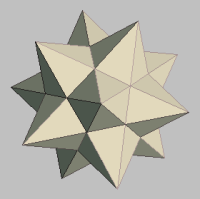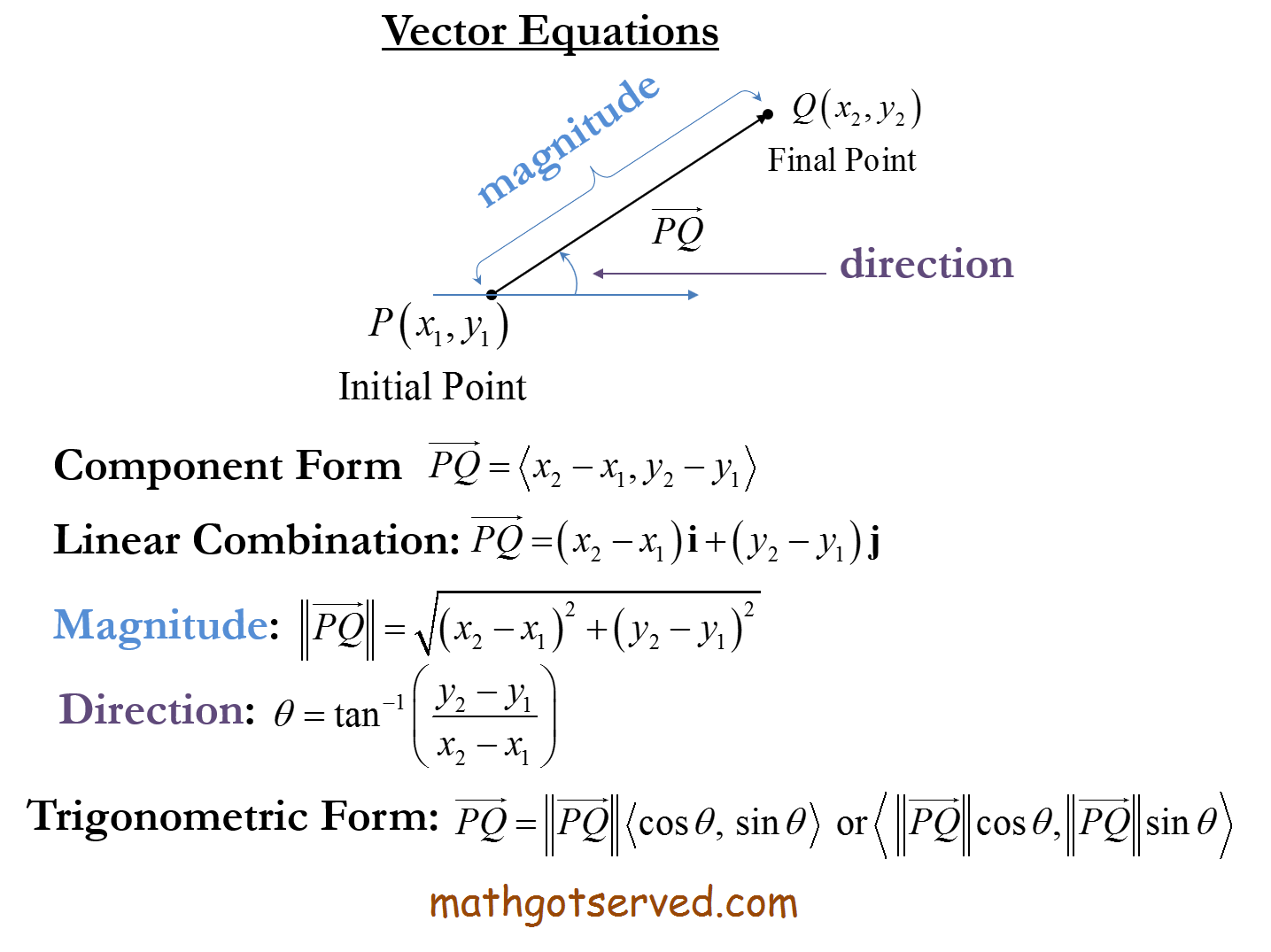MATHGOTSERVEDVECTORS
Vectors
Any quantity with magnitude and direction is known as a vector. Examples of vectors include velocity, and acceleration. Vectors are made up or two components the magnitude also known as a scalar quantity and the direction which simply specifies the direction in which the vector is acting. If a vector represents the speed and direction of a particle then it represents the velocity of the particle.

Vectors are represented by directed line segmetns. It starts from a point known as the initieal point and ends at the terminal point. The magnitude of the vector is the distance of the vector from the initial to the terminal point.

The Unit Vector is a vector with length or magnitude of 1. The unit vector of any vector can be found by dividing a vector by its magnitude.

If a vector has its initial point at the origin, it is in standard position.
Two vectors are equal if and only if they have the same magnitude and direction.
The sum of two or more vectors is called the resultant of the vectors. There are two methods for finding the resultant of two vectors. The Parallelogram method also known ans the tail to tail method, and the triangle method also known as the tip to tail method of vector resolution.